Saturday 17th April 2021

CBSE Guess > Papers > Question Papers > Class XII > 2006 > Mathematics > Outside Delhi Set - I.

Mathematics — 2006 (Set I — Outside Delhi)

SECTION – A

Q. 1. Express the matrix as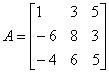the sum of a symmetric and a skew-symmetric matrix.

Q. 2. Using properties of determinants, prove the following: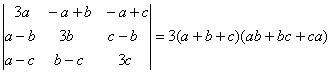Q. 3. Solve the following differentia equation: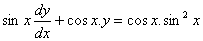Q. 4. Verify that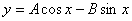is solution of the differential equation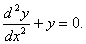Or

Solve the following differential equation:Q. 5.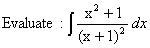Or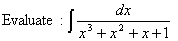Q. 6.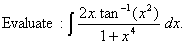Q. 7. Two cards are drawn successively with replacement from a well shuffled pack of 52 cards. Find the probability distribution of number of jacks.

Q. 8. A and B toss a coin alternately till one of them gets a head and wins the game. If A starts first, find the probability that B will win the game.

Q. 9. Shove that the following argument is in valid: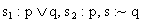Or

Construct an input/output table for the following circuit: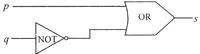Q. 10.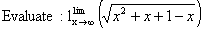Q. 11. Differentiate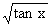w. r. t. x from first principle.

Q. 12.Or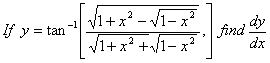Q. 13.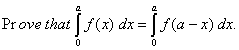Q. 14.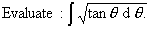Q. 15. Find the intervals in which the function f (x) = x3 - 12x2 + 36x + 17 is (a) increasing, (b) decreasing.

Q. 16. Using matrices, solve the following system of equations: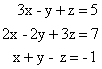Q. 17. An open box, with a square base, is to be made out of a given quantity of metal sheet of area C2. Show that the maximum volume of the box is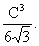Or

A window is in the form of a rectangle surmounted by a semi-circle. If the total perimeter of the window is3O m, find the dimensions of the window so that maximum light is admitted.

Q. 18. Find the area of the region bounded by the parabola x2 = 4y and the line x = 4y – 2.

Or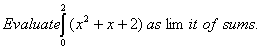SECTION – B

Q. 19. Find the angle between the vectors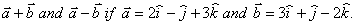Q. 20. Using vectors, prove that in a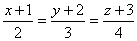where a, b and c are lengths of the sides opposite, respectively, to the angles A, B and C ofQ. 21. Find the coordinates of the point where the linemeets the plane x + y + 4z = 6.

Q. 22. Find the image of the point (1, 2, 3) in the plane x + 2y + 4z = 38.

Q. 23. Find the radius of the circular section of sphere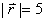by the plane.

Q. 24. The resultant of two forcesacting at an angle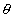is equal to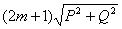and when they act an angle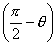, the resultant is equal to, Show that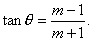Q. 25. A body of mass 10 kg hags by a string from a fixed point. The string is drawn out of the vertical by applying a force 49 N to the body. In which direction should this force be applied in order that, in equilibrium, the deflection of the string from the vertical may be of 300? Also find the tension in the string.

Q. 26. A particle is projected so as to graze the tops of two walls, each of height 10m, at distances 15 m and 45 m respectively from the point of projection. Find the angle of projection.

SECTION – C

Q. 19. In a bolt factory, machines A, B, C manufacture 25%, 35% and 40% respectively of the total bolts. Of their output 5%, 4% and 2% respectively are defective bolts. A bolt is drawn at random and is found to be defective. Find the probability that it is manufactured by machine B.

Q. 20. The mean and variance of the binomial distribution are 4 and 4/3 respectively. Find the distribution and

Q. 21. The banker’s discount and banker’s gain on a certain bill of exchange, due after a certain time, are respectively Rs.1,250 and Rs.50. Find the face value of the bill.

Q. 22. A bill for Rs.5,050 is drawn on April 13, 2005. It is discounted on July 4,2005 at 5% per annum. If the banker’s gain in the transaction is Rs. 0.50 find the nominal date of maturity of the bill.

Q. 23. A machine costs a company Rs 5,75,000 and its effective life is estimated to be 20 years. A sinking fund is created for replacing the machine at the end of its life-time when its scrap realises a sum of Rs.75,000 only. Calculate what amount should be provided every year out of profits, for the sinking fund if it accumulates an interest of 5% per annum, compounded annually. [Use(1.05)20 = 2.6551]

Q. 24. A and B are partners sharing profits and losses in the ratio 5 : 3. C pays Rs.96,000 as premium for the goodwill of the business and is admitted for 1/5th share. Show how this amount will be shared by A and B, assuming that the share of C is contributed by A and B in their profit sharing ratio. Find also the new profit sharing ratio.

Q. 25. The manufacturing cost of an item consists of Rs. 900 as overheads, the material costs Rs.3 per item and Iabour cost is x2/100 for x items produced. How many items must be produced to have minimum average cost?

Q. 26. David wants to invest at most Rs. 12,000 in bonds A and B. According to the rule, he has to invest at least Rs. 2,000 in Bond A and at least Rs.4,000 in Bond B. If the rates of interest on Bonds A and B respectively are 8% and 10% per annum, formulate the problem as L.P.P. and solve it graphically for maximum interest. Also determine the maximum interest received in a year.

 Mathematics 2006 Question Papers Class XII Delhi Outside Delhi Compartment Delhi Compartment Outside DelhiSet ISet ISet ISet ISet IISet IISet IISet IISet IIISet III

 CBSE 2006 Question Papers Class XIIEnglishSociologyFunctional EnglishPsychologyMathematicsPhilosopyPhysicsComputer ScienceChemistryEntrepreneurshipBiologyInformatics PracticesGeographyMultimedia & Web TechnologyEconomicsBiotechnologyBusiness StudiesPhysical EducationAccountancyFine ArtsPolitical ScienceHistoryAgriculture